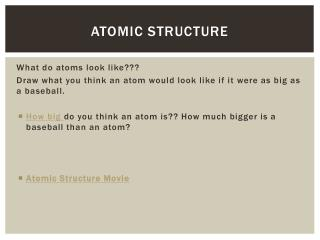Download PresentationAtomic Structure

Atomic Structure - PowerPoint PPT Presentation

Atomic Structure. What do atoms look like??? Draw what you think an atom would look like if it were as big as a baseball. How big do you think an atom is?? How much bigger is a baseball than an atom? Atomic Structure Movie. Atomic Structure Tool.I am the owner, or an agent authorized to act on behalf of the owner, of the copyrighted work described.
Download PresentationAtomic Structure

Download Policy: Content on the Website is provided to you AS IS for your information and personal use and may not be sold / licensed / shared on other websites without getting consent from its author.While downloading, if for some reason you are not able to download a presentation, the publisher may have deleted the file from their server.

- - - - - - - - - - - - - - - - - - - - - - - - - - E N D - - - - - - - - - - - - - - - - - - - - - - - - - -
Presentation Transcript
1. Atomic Structure What do atoms look like??? Draw what you think an atom would look like if it were as big as a baseball. • How big do you think an atom is?? How much bigger is a baseball than an atom? • Atomic Structure Movie

2. Atomic Structure Tool What happens when the # of particles changes? Experiment animation

3. Go To Phet…. • How do you Build an Atom ?

4. Average Atomic Mass: Isotopes •  Isotopes are forms of the same atom that vary in mass.  • There are two different types (isotopes) of copper atoms. One type of copper atoms weighs in at 62.93 amu, the other has a mass of 64.94 amu. The lighter isotope is more common with  69.09% of the naturally occurring copper having a mass of 62.93 amu per atom. The remainder of the atoms, 30.91 %, have a mass of 64.94 amu. • To find the AVERAGE ATOMIC MASS of an atom, we take into account all of the isotopes that exist and the percentage of each type. The calculation of the average atomic mass is a WEIGHTED AVERAGE. • Average atomic mass = Σ (mass of isotope × relative abundance)

5. Since there are two isotopes, so we will be adding the contributions of 2 isotopes. (That’s where the Σ sign comes in. ) The relative abundance is simply the percentage of the isotope, but in decimal format.  0.90% corresponds to a relative abundance of 0.6090. • Average atomic mass of copper = (62.93 amu × 0.6909) + (64.94 amu × 0.3091)= 63.55

6. Find the Average Mass of Silver • What’s the remaining percentage? ______________ • What the average mass of silver? _______________

7. Find the average mass of Silicon.. • Look over the data before you begin the problem. Estimate the value of the answer before you begin the calculation. Will the weighted average be closer to 28,  29, or 30?   • Calculate the average… How good was your guess?

8. Find the average mass of Iron • Make a Guess…. What do you think the answer is? _________ • Now, do the calculation– the average mass is ________

9. Some vocab to review • Atomic Mass • Isotope • Formula Mass • Molar Mass • Mass Number • Proton • Neutron • Electron • Average Atomic Mass

10. Absorption Spectrum • Bohr’s model and electrons absorbing energy

11. Electron Configurations The Principal Energy Level (the #) only holds that # of sublevels.

12. Number of Electrons per Sublevel

13. Overlap of Energy Levels

14. Writing Electron Configurations • Let’s try a few…. • Li = 1s22s1 • Boron =

15. Lewis Dot Structures • Kernal- Represented by the symbol. It is the nucleus and the inner electrons of an atom or ion • Valence Electrons- Represented by the dots. The electrons in the outer most energy level of an atom or ion.

16. Lewis Dot Diagrams: • Show the valence Electrons—the ones that make bonds • Oxygen gets 6 dots. • Chlorine gets 7 dots. NOTE****There is an order we fill the dots.

17. Practice THis • What do you think the Lewis Dot Diagram will be for these Elements? Write the Dot Diagram for each • Na • Be • Al • C • N • O • Cl • Ne • What do you notice about the Dot Diagram series you just made? What do you notice about the position of the elements on the periodic table?

18. What happens when there’s a different # of electrons • LDDs for Ions: • What’s the charge for Oxide? How did it get that charge? • What’s the charge for Aluminum (when it’s ionic)? What happened to the atom that it now has a charge? • What do you notice about the valence shell of these ions?